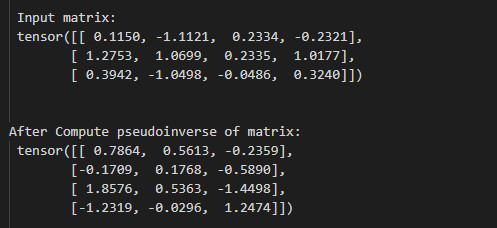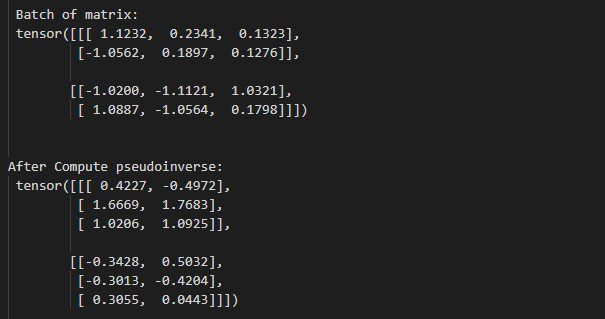Open in App
Not now

# How to Compute the Pseudoinverse of a Matrix in PyTorch

• Last Updated : 01 May, 2022

In this article, we are going to discuss how to compute the pseudoinverse of a matrix in Python using PyTorch.

## torch.linalg.pinv() method

torch.linalg.pinv() method accepts a matrix and a batch of matrices as input and returns a new tensor with the pseudoinverse of the input matrix. if the input is a batch of matrices then the output tensor also has the same batch dimensions. This method also supports the input of float, double, cfloat, and cdouble dtypes. The below syntax is used to compute the pseudoinverse of a matrix.

Syntax: torch.linalg.pinv(inp)

Parameters:

• inp: Where inp is a Matrix of Order M x N or a batch of matrices.

Returns: it will returns a new tensor with the pseudoinverse of the input matrix.

Example 1:

In this example, we will understand how to compute the pseudoinverse of a matrix in PyTorch.

## Python3

 `# import torch libraries ` `import` `torch ` ` `  `# creating a matrix or 4x3 ` `inp ``=` `torch.tensor([[``0.1150``, ``-``1.1121``,  ` `                     ``0.2334``, ``-``0.2321``], ` `                    ``[``1.2753``, ``1.0699``, ` `                     ``0.2335``, ``1.0177``], ` `                    ``[``0.3942``, ``-``1.0498``,  ` `                     ``-``0.0486``, ``0.3240``]]) ` ` `  `# Display matrix ` `print``(``"\n Input matrix: \n"``, inp) ` ` `  `# compute pseudoinverse of above defined matrix ` `output ``=` `torch.linalg.pinv(inp) ` ` `  `# Display result ` `print``(``"\n After Compute pseudoinverse of matrix: \n"``, ` `      ``output) `

Output:Example 2:

In this example, we will compute the pseudoinverse of a batch of matrices in PyTorch.

## Python3

 `# import torch libraries ` `import` `torch ` ` `  `# creating a batch matrix ` `inp ``=` `torch.tensor([[[``1.1232``, ``0.2341``, ``0.1323``], ` `                     ``[``-``1.0562``, ``0.1897``, ``0.1276``]], ` `                    ``[[``-``1.0200``, ``-``1.1121``, ``1.0321``], ` `                     ``[``1.0887``, ``-``1.0564``, ``0.1798``]]]) ` ` `  `# Display batch of matrix ` `print``(``"\n Batch of matrix: \n"``, inp) ` ` `  `# compute pseudoinverse of above defined matrix ` `output ``=` `torch.linalg.pinv(inp) ` ` `  `# Display result ` `print``(``"\n After Compute pseudoinverse: \n"``, ` `      ``output) `

Output:My Personal Notes arrow_drop_up
Related Articles